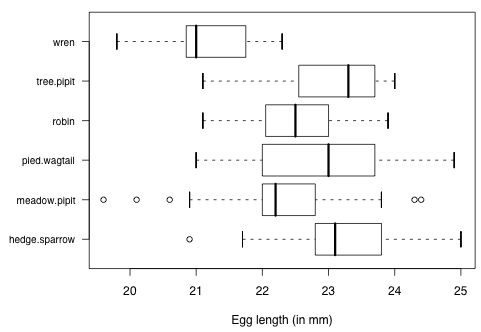2404 Identify Bivariate Displays

15 Questions | Total Attempts: 145Settings• 1.
The image above displays bivariate data as a :
• A.

Parallel Box & Whisker Plot

• B.

Back to Back Stem Plot

• C.

Two Way Frequency Table

• D.

Parallel Segmented Bar Charts

• E.

Scatter Plot

• F.

Not a Bivariate Display

• 2.
The image above displays bivariate data as a :
• A.

Parallel Box & Whisker Plot

• B.

Back to Back Stem Plot

• C.

Two Way Frequency Table

• D.

Parallel Segmented Bar Charts

• E.

Scatter Plot

• F.

Not a Bivariate Display

• 3.
The image above displays bivariate data as a :
• A.

Parallel Box & Whisker Plot

• B.

Back to Back Stem Plot

• C.

Two Way Frequency Table

• D.

Parallel Segmented Bar Charts

• E.

Scatter Plot

• F.

Not a Bivariate Display

• 4.
The image above displays bivariate data as a :
• A.

Parallel Box & Whisker Plot

• B.

Back to Back Stem Plot

• C.

Two Way Frequency Table

• D.

Parallel Segmented Bar Charts

• E.

Scatter Plot

• F.

Not a Bivariate Display

• 5.
The image above displays bivariate data as a :
• A.

Parallel Box & Whisker Plot

• B.

Back to Back Stem Plot

• C.

Two Way Frequency Table

• D.

Parallel Segmented Bar Charts

• E.

Scatter Plot

• F.

Not a Bivariate Display

• 6.
The image above displays bivariate data as a :
• A.

Parallel Box & Whisker Plot

• B.

Back to Back Stem Plot

• C.

Two Way Frequency Table

• D.

Parallel Segmented Bar Charts

• E.

Scatter Plot

• F.

Not a Bivariate Display

• 7.
The image above displays bivariate data as a :
• A.

Parallel Box & Whisker Plot

• B.

Back to Back Stem Plot

• C.

Two Way Frequency Table

• D.

Parallel Segmented Bar Charts

• E.

Scatter Plot

• F.

Not a Bivariate Display

• 8.
The image above displays bivariate data as a :
• A.

Parallel Box & Whisker Plot

• B.

Back to Back Stem Plot

• C.

Two Way Frequency Table

• D.

Parallel Segmented Bar Charts

• E.

Scatter Plot

• F.

Not a Bivariate Display

• 9.
The image above displays bivariate data as a :
• A.

Parallel Box & Whisker Plot

• B.

Back to Back Stem Plot

• C.

Two Way Frequency Table

• D.

Parallel Segmented Bar Charts

• E.

Scatter Plot

• F.

Not a Bivariate Display

• 10.
The image above displays bivariate data as a :
• A.

Parallel Box & Whisker Plot

• B.

Back to Back Stem Plot

• C.

Two Way Frequency Table

• D.

Parallel Segmented Bar Charts

• E.

Scatter Plot

• F.

Not a Bivariate Display

• 11.